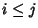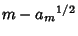## B2-Sequence

N.B. A detailed on-line essay by S. Finch was the starting point for this entry.

Also called a Sidon Sequence. An Infinite Sequence of Positive Integers(1)

such that all pairwise sums(2)

forare distinct (Guy 1994). An example is 1, 2, 4, 8, 13, 21, 31, 45, 66, 81, ... (Sloane's A005282).

Zhang (1993, 1994) showed that(3)

The definition can be extended to-sequences (Guy 1994).

References

Finch, S. Favorite Mathematical Constants.'' http://www.mathsoft.com/asolve/constant/erdos/erdos.html

Guy, R. K. Packing Sums of Pairs,'' Three-Subsets with Distinct Sums,'' and-Sequences,'' and-Sequences Formed by the Greedy Algorithm.'' §C9, C11, E28, and E32 in Unsolved Problems in Number Theory, 2nd ed. New York: Springer-Verlag, pp. 115-118, 121-123, 228-229, and 232-233, 1994.

Sloane, N. J. A. Sequence A005282/M1094 in An On-Line Version of the Encyclopedia of Integer Sequences.'' http://www.research.att.com/~njas/sequences/eisonline.html and Sloane, N. J. A. and Plouffe, S. The Encyclopedia of Integer Sequences. San Diego: Academic Press, 1995.

Zhang, Z. X. A B2-Sequence with Larger Reciprocal Sum.'' Math. Comput. 60, 835-839, 1993.

Zhang, Z. X. Finding Finite B2-Sequences with Larger.'' Math. Comput. 63, 403-414, 1994.